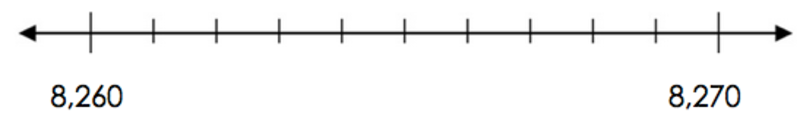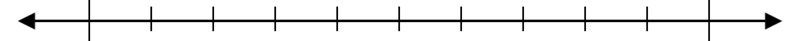# Rounding on the Number Line

Alignments to Content Standards: 4.NBT.A.3

1. The number 8,263 lies between 8,260 and 8,270 on the number line.  Label all the other tick marks between 8,260 and 8,270. Is 8,263 closer to 8,260 or 8,270 on the number line?2. Which hundred is 8,263 nearest to on the number line?  Plot 8,200 and 8,300 on the two outermost spots on the number line below.  Then plot 8,263 to prove your answer.3. Which thousand is 8,263 nearest to on the number line?  Plot 8,000 and 9,000 on the two outermost spots on the number line below.  Then plot 8,263 to prove your answer.## IM Commentary

This task helps students understand the concept of rounding by using the number line to illustrate how to find the closest benchmark numbers.  This task also helps students lay the groundwork for developing more efficient rules for rounding based on the digits in a given number.

As students reason through the value of each tick mark on each number line, teachers should engage in formative assessment questioning to check that students understand what one tenth of each interval must be as the interval changes throughout this question.  It will be natural for students to try initial values that may be wrong for each increment, so when possible let them test out their own predictions and learn to correct their own misunderstandings.  As they count up by that unit on their number line, they should realize their mistakes as they see the distance between each number on their number line will not be equal.

This task has a related and more rigorous 5th grade version: https://www.illustrativemathematics.org/illustrations/1804​.

## Solution

part a.

8,263 is closest to 8,260.  In other words, 8,263 rounded to the nearest ten is 8,260.part b.

8,263 is closest to 8,300.  In other words, 8,263 rounded to the nearest hundred is 8,300.part c.

8,263 is closest to 8,000.  In other words, 8,263 rounded to the nearest thousand is 8,000.